# plot

Plot receiver operating characteristic (ROC) curves and other performance curves

Since R2022a

## Syntax

``plot(rocObj)``
``plot(ax,rocObj)``
``plot(___,Name=Value)``
``curveObj = plot(___)``
``[curveObj,graphicsObjs] = plot(___)``

## Description

example

````plot(rocObj)` creates a receiver operating characteristic (ROC) curve, which is a plot of the true positive rate (TPR) versus the false positive rate (FPR), for each class in the `ClassNames` property of the `rocmetrics` object `rocObj`. The function marks the model operating point for each curve, and displays the value of the area under the ROC curve (AUC) and the class name for the curve in the legend.```
``plot(ax,rocObj)` creates the plot on the axes specified by `ax` instead of the current axes.`

example

``plot(___,Name=Value)` specifies additional options using one or more name-value arguments in addition to any of the input argument combinations in the previous syntaxes. For example, `AverageROCType="macro",ClassNames=[]` computes the average performance metrics using the macro-averaging method and plots the average ROC curve only.`

example

``curveObj = plot(___)` returns a `ROCCurve` object for each performance curve.`
``[curveObj,graphicsObjs] = plot(___)` also returns graphics objects for the model operating points and diagonal line.`

## Examples

collapse all

Create a `rocmetrics` object for a multiclass classification problem, and plot a ROC curve for each class.

Load the `fisheriris` data set. The matrix `meas` contains flower measurements for 150 different flowers. The vector `species` lists the species for each flower. `species` contains three distinct flower names.

`load fisheriris`

Train a classification tree that classifies observations into one of the three labels. Cross-validate the model using 10-fold cross-validation.

```rng("default") % For reproducibility Mdl = fitctree(meas,species,Crossval="on");```

Compute the classification scores for validation-fold observations.

```[~,Scores] = kfoldPredict(Mdl); size(Scores)```
```ans = 1×2 150 3 ```

`Scores` is a matrix of size `150`-by-`3`. The column order of `Scores` follows the class order in `Mdl`. Display the class order stored in `Mdl.ClassNames`.

`Mdl.ClassNames`
```ans = 3x1 cell {'setosa' } {'versicolor'} {'virginica' } ```

Create a `rocmetrics` object by using the true labels in `species` and the classification scores in `Scores`. Specify the column order of `Scores` using `Mdl.ClassNames`.

`rocObj = rocmetrics(species,Scores,Mdl.ClassNames);`

Plot the ROC curve for each class.

`plot(rocObj)`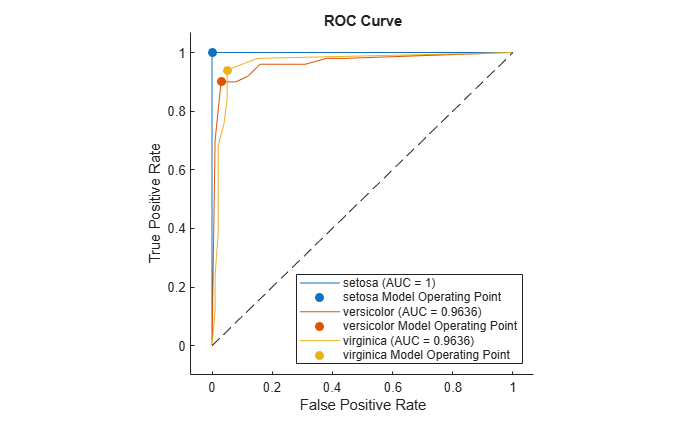For each class, the `plot` function plots a ROC curve and displays a filled circle marker at the model operating point. The legend displays the class name and AUC value for each curve.

Plot the average ROC curve by using the `plot` function. Use a `ROCCurve` object, an output of the `plot` function, to obtain the average metric values.

Load the `fisheriris` data set. The matrix `meas` contains flower measurements for 150 different flowers. The vector `species` lists the species for each flower. `species` contains three distinct flower names.

`load fisheriris`

Train a classification tree that classifies observations into one of the three labels. Cross-validate the model using 10-fold cross-validation.

```rng("default") % For reproducibility Mdl = fitctree(meas,species,Crossval="on");```

Compute the classification scores for validation-fold observations.

`[~,Scores] = kfoldPredict(Mdl);`

Create a `rocmetrics` object.

`rocObj = rocmetrics(species,Scores,Mdl.ClassNames);`

Plot the ROC curve for each class. Specify `AverageROCType="macro"` to compute metrics for the average ROC curve using the macro-averaging method.

`curveObj = plot(rocObj,AverageROCType="macro")`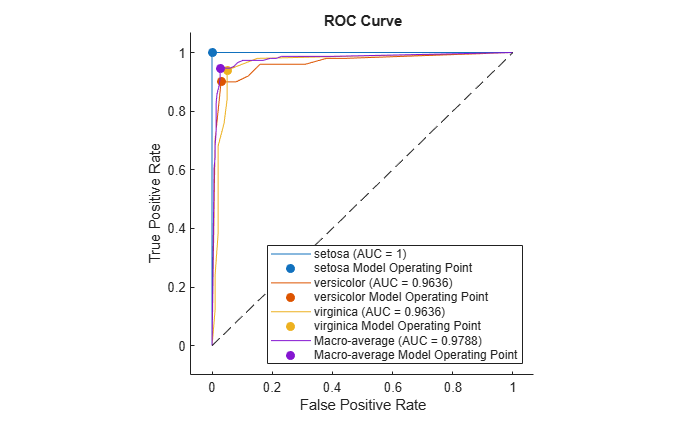```curveObj = 4x1 ROCCurve array: ROCCurve (setosa (AUC = 1)) ROCCurve (versicolor (AUC = 0.9636)) ROCCurve (virginica (AUC = 0.9636)) ROCCurve (Macro-average (AUC = 0.9788)) ```

The `plot` function returns a `ROCCurve` object for each performance curve. You can use the object to query and set properties of the plot after creating it.

Display the data points of the average ROC curve stored in the fourth element of `curveObj`.

```tbl_average = table(curveObj(4).Thresholds,curveObj(4).XData,curveObj(4).YData, ... VariableNames=["Threshold",curveObj(4).XAxisMetric,curveObj(4).YAxisMetric])```
```tbl_average=32×3 table Threshold FalsePositiveRate TruePositiveRate _________ _________________ ________________ 1 0 0 1 0.0066667 0.60667 0.95455 0.01 0.64 0.95349 0.01 0.68 0.95238 0.013333 0.72667 0.95122 0.013333 0.82667 0.91304 0.016667 0.86 0.91111 0.023333 0.88667 0.86957 0.026667 0.91333 0.6 0.026667 0.92667 0.33333 0.026667 0.94 0.2 0.026667 0.94667 -0.2 0.03 0.94667 -0.33333 0.036667 0.94667 -0.6 0.046667 0.94667 -0.66667 0.053333 0.94667 ⋮ ```

Create a `rocmetrics` object and plot performance curves by using the `plot` function. Specify the `XAxisMetric` and `YAxisMetric` name-value arguments of the `plot` function to plot different types of performance curves other than the ROC curve. If you specify new metrics when you call the `plot` function, the function computes the new metrics and then uses them to plot the curve.

Load the `ionosphere` data set. This data set has 34 predictors (`X`) and 351 binary responses (`Y`) for radar returns, either bad (`'b'`) or good (`'g'`).

`load ionosphere`

Partition the data into training and test sets. Use approximately 80% of the observations to train a support vector machine (SVM) model, and 20% of the observations to test the performance of the trained model on new data. Partition the data using `cvpartition`.

```rng("default") % For reproducibility of the partition c = cvpartition(Y,Holdout=0.20); trainingIndices = training(c); % Indices for the training set testIndices = test(c); % Indices for the test set XTrain = X(trainingIndices,:); YTrain = Y(trainingIndices); XTest = X(testIndices,:); YTest = Y(testIndices);```

Train an SVM classification model.

`Mdl = fitcsvm(XTrain,YTrain);`

Compute the classification scores for the test set.

`[~,Scores] = predict(Mdl,XTest);`

Create a `rocmetrics` object. The `rocmetrics` function computes the FPR and TPR at different thresholds.

`rocObj = rocmetrics(YTest,Scores,Mdl.ClassNames);`

Plot the precision-recall curve for the first class. Specify the y-axis metric as precision (or positive predictive value) and the x-axis metric as recall (or true positive rate). The `plot` function computes the new metric values and plots the curve. Display a filled circle at the model operating point. Also, compute the area under the precision-recall curve using the trapezoidal method of the `trapz` function, and display the value in the legend.

```curveObj = plot(rocObj,ClassNames=Mdl.ClassNames(1), ... YAxisMetric="PositivePredictiveValue",XAxisMetric="TruePositiveRate"); hold on idx = find(curveObj.Thresholds>=0,1,'last'); scatter(curveObj.XData(idx),curveObj.YData(idx),[],curveObj.Color,"filled") xyData = rmmissing([curveObj.XData curveObj.YData]); auc = trapz(xyData(:,1),xyData(:,2)); legend(join([string(Mdl.ClassNames(1)) " (AUC = " string(auc) ")"],""), ... join([string(Mdl.ClassNames(1)) "Model Operating Point"]), ... Location="southwest") xlabel("Recall") ylabel("Precision") title("Precision-Recall Curve") hold off```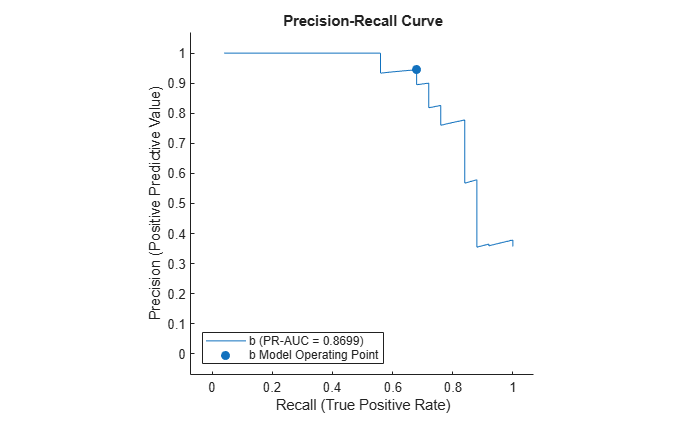Plot the detection error tradeoff (DET) graph for the first class. Specify the y-axis metric as the false negative rate and the x-axis metric as the false positive rate. Use a log scale for the x-axis and y-axis.

```f = figure; plot(rocObj,ClassNames=Mdl.ClassNames(1), ... YAxisMetric="FalseNegativeRate",XAxisMetric="FalsePositiveRate") f.CurrentAxes.XScale = "log"; f.CurrentAxes.YScale = "log"; title("DET Graph")```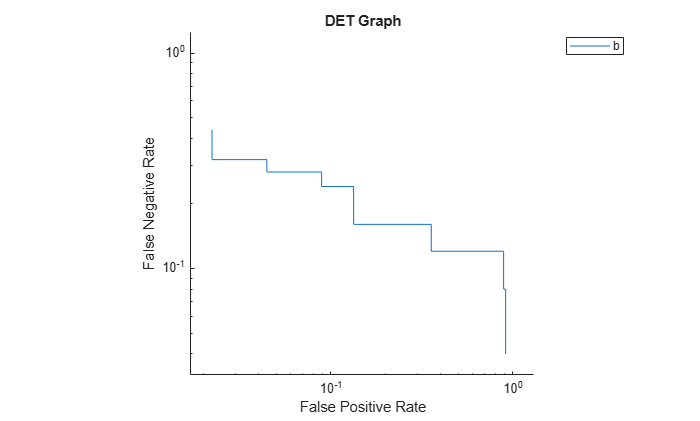Compute the confidence intervals for FPR and TPR for fixed threshold values by using bootstrap samples, and plot the confidence intervals for TPR on the ROC curve.

Load the `fisheriris` data set. The matrix `meas` contains flower measurements for 150 different flowers. The vector `species` lists the species for each flower. `species` contains three distinct flower names.

`load fisheriris`

Train a naive Bayes model that classifies observations into one of the three labels. Cross-validate the model using 10-fold cross-validation.

```rng("default") % For reproducibility Mdl = fitcnb(meas,species,Crossval="on");```

Compute the classification scores for validation-fold observations.

`[~,Scores] = kfoldPredict(Mdl);`

Create a `rocmetrics` object. Specify `NumBootstraps` as 100 to use 100 bootstrap samples to compute the confidence intervals.

```rocObj = rocmetrics(species,Scores,Mdl.ClassNames, ... NumBootstraps=100);```

Plot the ROC curve and the confidence intervals for TPR. Specify `ShowConfidenceIntervals=true` to show the confidence intervals.

`plot(rocObj,ShowConfidenceIntervals=true)`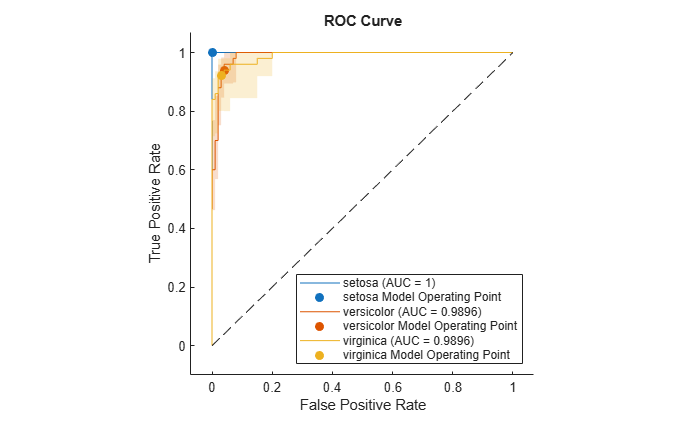The shaded area around each curve indicates the confidence intervals. The widths of the confidence intervals for `setosa` are 0 for nonzero false positive rates, so the plot does not have a shaded area for `setosa`.

`rocmetrics` computes the ROC curves from the cross-validated scores. Therefore, each ROC curve represents an estimate of a ROC curve on unseen test data for a model trained on the full data set (`meas` and `species`). The confidence intervals represent the estimates of uncertainty for the curve. This uncertainty is due to the variance in unseen test data for the model trained on the full data set.

Compute the performance metrics (FPR and TPR) for a binary classification problem by creating a `rocmetrics` object, and plot a ROC curve by using the `plot` function. The `plot` function displays a filled circle at the model operating point. Display a data tip at the model operating point.

Load the `ionosphere` data set. This data set has 34 predictors (`X`) and 351 binary responses (`Y`) for radar returns, either bad (`'b'`) or good (`'g'`).

`load ionosphere`

Partition the data into training and test sets. Use approximately 80% of the observations to train a support vector machine (SVM) model, and 20% of the observations to test the performance of the trained model on new data. Partition the data using `cvpartition`.

```rng("default") % For reproducibility of the partition c = cvpartition(Y,Holdout=0.20); trainingIndices = training(c); % Indices for the training set testIndices = test(c); % Indices for the test set XTrain = X(trainingIndices,:); YTrain = Y(trainingIndices); XTest = X(testIndices,:); YTest = Y(testIndices);```

Train an SVM classification model.

`Mdl = fitcsvm(XTrain,YTrain);`

Compute the classification scores for the test set.

`[~,Scores] = predict(Mdl,XTest);`

Create a `rocmetrics` object.

`rocObj = rocmetrics(YTest,Scores,Mdl.ClassNames);`

The `rocmetrics` function computes the FPR and TPR at different thresholds and finds the AUC value.

Plot the ROC curve. Specify `ClassNames` to plot the curve for the first class.

`curveObj = plot(rocObj,ClassNames=Mdl.ClassNames(1));`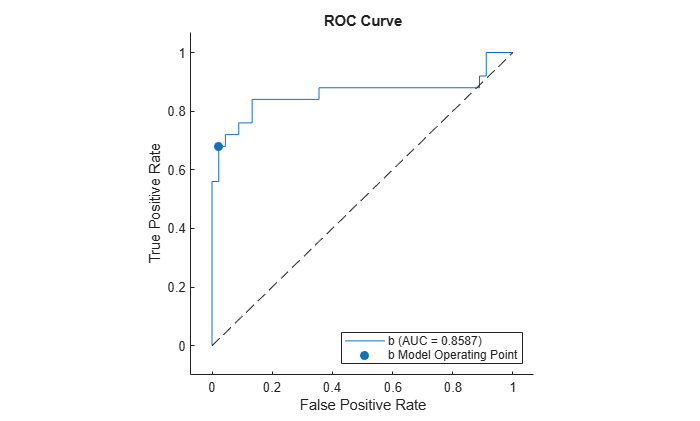The `plot` function returns a `ROCCurve` object for each performance curve. You can use the object to query and set the properties of the plot after creating it.

The filled circle marker indicates the model operating point at which the threshold value is 0. The function chooses a point that has the largest threshold value less than or equal to 0. The legend displays the class name and AUC value for the curve.

You can create data tips by clicking data points on the curve. Alternatively, you can create data tips using the `datatip` function.

Find the model operating point in the `Metrics` property of `rocObj` for class `b`. The `predict` function classifies an observation into the class yielding a larger score, which corresponds to the class with a nonnegative adjusted score. That is, the typical threshold value used by the `predict` function is 0. Among the rows in the `Metrics` property of `rocObj` for class `b`, find the point that has the smallest nonnegative threshold value. The point on the curve indicates identical performance to the performance of the threshold value 0.

```idx_b = strcmp(rocObj.Metrics.ClassName,"b"); t = rocObj.Metrics(idx_b,:); X = rocObj.Metrics(idx_b,:).FalsePositiveRate; Y = rocObj.Metrics(idx_b,:).TruePositiveRate; T = rocObj.Metrics(idx_b,:).Threshold; idx_model = find(T>=0,1,"last"); modelpt = [T(idx_model) X(idx_model) Y(idx_model)]```
```modelpt = 1×3 0.1045 0.0222 0.6800 ```

Display a data tip at the model operating point. Specify the target graph object as the output object of the `plot` function.

`datatip(curveObj,DataIndex=idx_model,Location="southeast");`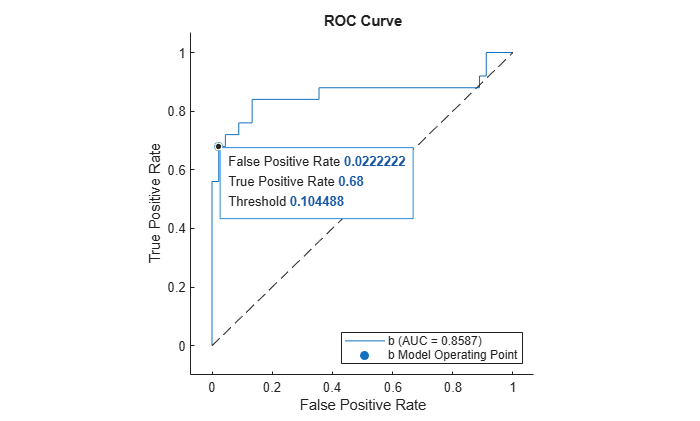## Input Arguments

collapse all

Object evaluating classification performance, specified as a `rocmetrics` object.

Target axes, specified as an `Axes` object.

If you do not specify the axes and the current axes are Cartesian, then `plot` uses the current axes (`gca`). For more information on creating an `Axes` object, see `axes` and `gca`.

### Name-Value Arguments

Specify optional pairs of arguments as `Name1=Value1,...,NameN=ValueN`, where `Name` is the argument name and `Value` is the corresponding value. Name-value arguments must appear after other arguments, but the order of the pairs does not matter.

Example: `YAxisMetric="PositivePredictiveValue",XAxisMetric="TruePositiveRate"` plots the precision (positive predictive value) versus the recall (true positive rate), which represents a precision-recall curve.

Method for averaging ROC curves, specified as `"none"`, `"micro"`, `"macro"`, `"weighted"`, a string array of method names, or a cell array of method names.

• If you specify `"none"` (default), the `plot` function does not create the average ROC curve. The `AverageROCType` value must be `"none"` if `plot` creates performance curves other than a ROC curve.

• If you specify multiple methods as a string array or a cell array of character vectors, then the `plot` function plots multiple average ROC curves using the specified methods.

• If you specify one or more averaging methods and specify `ClassNames=[]`, then the `plot` function plots only the average ROC curves.

`plot` computes the averages of performance metrics for a multiclass classification problem, and plots the average ROC curves using these methods:

• `"micro"` (micro-averaging) — `plot` finds the average performance metrics by treating all one-versus-all binary classification problems as one binary classification problem. The function computes the confusion matrix components for the combined binary classification problem, and then computes the average FPR and TPR using the values of the confusion matrix.

• `"macro"` (macro-averaging) — `plot` computes the average values for FPR and TPR by averaging the values of all one-versus-all binary classification problems.

• `"weighted"` (weighted macro-averaging) — `plot` computes the weighted average values for FPR and TPR using the macro-averaging method and using the prior class probabilities (the `Prior` property of `rocObj`) as weights.

The algorithm type determines the length of the vectors in the `XData`, `YData`, and `Thresholds` properties of a `ROCCurve` object, returned by `plot`, for the average ROC curve. For more details, see Average of Performance Metrics.

Example: `AverageROCType="macro"`

Example: `AverageROCType=["micro","macro"]`

Data Types: `char` | `string`

Class labels to plot, specified as a categorical, character, or string array, logical or numeric vector, or cell array of character vectors. The values and data types in `ClassNames` must match those of the class names in the `ClassNames` property of `rocObj`. (The software treats character or string arrays as cell arrays of character vectors.)

• If you specify multiple class labels, the `plot` function plots a ROC curve for each class.

• If you specify `ClassNames=[]` and specify one or more averaging methods using `AverageROCType`, then the `plot` function plots only the average ROC curves.

Example: `ClassNames=["red","blue"]`

Data Types: `single` | `double` | `logical` | `char` | `string` | `cell` | `categorical`

Flag to show the confidence intervals of the y-axis metric (`YAxisMetric`), specified as logical `0` (`false`) or `1` (`true`).

The `ShowConfidenceIntervals` value can be `true` only if the `Metrics` property of `rocObj` contains the confidence intervals for the y-axis metric.

Example: `ShowConfidenceIntervals=true`

Data Types: `logical`

Flag to show the diagonal line that extends from `[0,0]` to `[1,1]`, specified as logical `1` (`true`) or `0` (`false`).

The default value is `true` if you plot a ROC curve or an average ROC curve, and `false` otherwise.

In the ROC curve plot, the diagonal line represents a random classifier, and the line passing through `[0,0]`, `[0,1]`, and `[1,1]` represents a perfect classifier.

Example: `ShowDiagonalLine=false`

Data Types: `logical`

Flag to show the model operating point, specified as logical `1` (`true`) or `0` (`false`).

The default value is `true` for a ROC curve, and `false` for an average ROC curve. The `ShowModelOperatingPoint` value must be false for performance curves other than ROC.

Example: `ShowModelOperatingPoint=false`

Data Types: `logical`

Metric for the x-axis, specified as a character vector or string scalar of the built-in metric name or a custom metric name, or a function handle (`@metricName`).

• Built-in metrics — Specify one of the following built-in metric names by using a character vector or string scalar.

NameDescription
`"TruePositives"` or `"tp"`Number of true positives (TP)
`"FalseNegatives"` or `"fn"`Number of false negatives (FN)
`"FalsePositives"` or `"fp"`Number of false positives (FP)
`"TrueNegatives"` or `"tn"`Number of true negatives (TN)
`"SumOfTrueAndFalsePositives"` or `"tp+fp"`Sum of TP and FP
`"RateOfPositivePredictions"` or `"rpp"`Rate of positive predictions (RPP), `(TP+FP)/(TP+FN+FP+TN)`
`"RateOfNegativePredictions"` or `"rnp"`Rate of negative predictions (RNP), `(TN+FN)/(TP+FN+FP+TN)`
`"Accuracy"` or `"accu"`Accuracy, `(TP+TN)/(TP+FN+FP+TN)`
`"TruePositiveRate"` or `"tpr"`True positive rate (TPR), also known as recall or sensitivity, `TP/(TP+FN)`
`"FalseNegativeRate"`, `"fnr"`, or `"miss"`False negative rate (FNR), or miss rate, `FN/(TP+FN)`
`"FalsePositiveRate"` or `"fpr"`False positive rate (FPR), also known as fallout or 1-specificity, `FP/(TN+FP)`
`"TrueNegativeRate"`, `"tnr"`, or `"spec"`True negative rate (TNR), or specificity, `TN/(TN+FP)`
`"PositivePredictiveValue"`, `"ppv"`, or `"prec"`Positive predictive value (PPV), or precision, `TP/(TP+FP)`
`"NegativePredictiveValue"` or `"npv"`Negative predictive value (NPV), `TN/(TN+FN)`
`"ExpectedCost"` or `"ecost"`

Expected cost, `(TP*cost(P|P)+FN*cost(N|P)+FP*cost(P|N)+TN*cost(N|N))/(TP+FN+FP+TN)`, where `cost` is a 2-by-2 misclassification cost matrix containing `[0,cost(N|P);cost(P|N),0]`. `cost(N|P)` is the cost of misclassifying a positive class (`P`) as a negative class (`N`), and `cost(P|N)` is the cost of misclassifying a negative class as a positive class.

The software converts the `K`-by-`K` matrix specified by the `Cost` name-value argument of `rocmetrics` to a 2-by-2 matrix for each one-versus-all binary problem. For details, see Misclassification Cost Matrix.

The software computes the scale vector using the prior class probabilities (`Prior`) and the number of classes in `Labels`, and then scales the performance metrics according to this scale vector. For details, see Performance Metrics.

• Custom metric stored in the `Metrics` property — Specify the name of a custom metric stored in the `Metrics` property of the input object `rocObj`. The `rocmetrics` function names a custom metric `"CustomMetricN"`, where `N` is the number that refers to the custom metric. For example, specify `XAxisMetric="CustomMetric1"` to use the first custom metric in `Metrics` as a metric for the x-axis.

• Custom metric — Specify a new custom metric by using a function handle. A custom function that returns a performance metric must have this form:

`metric = customMetric(C,scale,cost)`

• The output argument `metric` is a scalar value.

• A custom metric is a function of the confusion matrix (`C`), scale vector (`scale`), and cost matrix (`cost`). The software finds these input values for each one-versus-all binary problem. For details, see Performance Metrics.

• `C` is a `2`-by-`2` confusion matrix consisting of `[TP,FN;FP,TN]`.

• `scale` is a `2`-by-`1` scale vector.

• `cost` is a `2`-by-`2` misclassification cost matrix.

The `plot` function names a custom metric `"Custom Metric"` for the axis label.

The software does not support cross-validation for a custom metric. Instead, you can specify to use bootstrap when you create a `rocmetrics` object.

If you specify a new metric instead of one in the `Metrics` property of the input object `rocObj`, the `plot` function computes and plots the metric values. If you compute confidence intervals when you create `rocObj`, the `plot` function also computes confidence intervals for the new metric.

The `plot` function ignores `NaN`s in the performance metric values. Note that the positive predictive value (PPV) is `NaN` for the reject-all threshold for which `TP` = `FP` = `0`, and the negative predictive value (NPV) is `NaN` for the accept-all threshold for which `TN` = `FN` = `0`. For more details, see Thresholds, Fixed Metric, and Fixed Metric Values.

Example: `XAxisMetric="FalseNegativeRate"`

Data Types: `char` | `string` | `function_handle`

Metric for the y-axis, specified as a character vector or string scalar of the built-in metric name or custom metric name, or a function handle (`@metricName`). For details, see `XAxisMetric`.

Example: `YAxisMetric="FalseNegativeRate"`

Data Types: `char` | `string` | `function_handle`

## Output Arguments

collapse all

Object for the performance curve, returned as a `ROCCurve` object or an array of `ROCCurve` objects. `plot` returns a `ROCCurve` object for each performance curve.

Use `curveObj` to query and modify properties of the plot after creating it. For a list of properties, see ROCCurve Properties.

Graphics objects for the model operating points and diagonal line, returned as a graphics array containing `Scatter` and `Line` objects.

`graphicsObjs` contains a `Scatter` object for each model operating point (if `ShowModelOperatingPoint=true`) and a `Line` object for the diagonal line (if `ShowDiagonalLine=true`). Use `graphicsObjs` to query and modify properties of the model operating points and diagonal line after creating the plot. For a list of properties, see Scatter Properties and Line Properties.

collapse all

### Receiver Operating Characteristic (ROC) Curve

A ROC curve shows the true positive rate versus the false positive rate for different thresholds of classification scores.

The true positive rate and the false positive rate are defined as follows:

• True positive rate (TPR), also known as recall or sensitivity — `TP/(TP+FN)`, where TP is the number of true positives and FN is the number of false negatives

• False positive rate (FPR), also known as fallout or 1-specificity — `FP/(TN+FP)`, where FP is the number of false positives and TN is the number of true negatives

Each point on a ROC curve corresponds to a pair of TPR and FPR values for a specific threshold value. You can find different pairs of TPR and FPR values by varying the threshold value, and then create a ROC curve using the pairs. For each class, `rocmetrics` uses all distinct adjusted score values as threshold values to create a ROC curve.

For a multiclass classification problem, `rocmetrics` formulates a set of one-versus-all binary classification problems to have one binary problem for each class, and finds a ROC curve for each class using the corresponding binary problem. Each binary problem assumes one class as positive and the rest as negative.

For a binary classification problem, if you specify the classification scores as a matrix, `rocmetrics` formulates two one-versus-all binary classification problems. Each of these problems treats one class as a positive class and the other class as a negative class, and `rocmetrics` finds two ROC curves. Use one of the curves to evaluate the binary classification problem.

For more details, see ROC Curve and Performance Metrics.

### Area Under ROC Curve (AUC)

The area under a ROC curve (AUC) corresponds to the integral of a ROC curve (TPR values) with respect to FPR from `FPR` = `0` to `FPR` = `1`.

The AUC provides an aggregate performance measure across all possible thresholds. The AUC values are in the range `0` to `1`, and larger AUC values indicate better classifier performance.

### One-Versus-All (OVA) Coding Design

The one-versus-all (OVA) coding design reduces a multiclass classification problem to a set of binary classification problems. In this coding design, each binary classification treats one class as positive and the rest of the classes as negative. `rocmetrics` uses the OVA coding design for multiclass classification and evaluates the performance on each class by using the binary classification that the class is positive.

For example, the OVA coding design for three classes formulates three binary classifications:

Each row corresponds to a class, and each column corresponds to a binary classification problem. The first binary classification assumes that class 1 is a positive class and the rest of the classes are negative. `rocmetrics` evaluates the performance on the first class by using the first binary classification problem.

### Model Operating Point

The model operating point represents the FPR and TPR corresponding to the typical threshold value.

The typical threshold value depends on the input format of the `Scores` argument (classification scores) specified when you create a `rocmetrics` object:

• If you specify `Scores` as a matrix, `rocmetrics` assumes that the values in `Scores` are the scores for a multiclass classification problem and uses adjusted score values. A multiclass classification model classifies an observation into a class that yields the largest score, which corresponds to a nonnegative score in the adjusted scores. Therefore, the threshold value is `0`.

• If you specify `Scores` as a column vector, `rocmetrics` assumes that the values in `Scores` are posterior probabilities of the class specified in `ClassNames`. A binary classification model classifies an observation into a class that yields a higher posterior probability, that is, a posterior probability greater than `0.5`. Therefore, the threshold value is `0.5`.

For a binary classification problem, you can specify `Scores` as a two-column matrix or a column vector. However, if the classification scores are not posterior probabilities, you must specify `Scores` as a matrix. A binary classifier classifies an observation into a class that yields a larger score, which is equivalent to a class that yields a nonnegative adjusted score. Therefore, if you specify `Scores` as a matrix for a binary classifier, `rocmetrics` can find a correct model operating point using the same scheme that it applies to a multiclass classifier. If you specify classification scores that are not posterior probabilities as a vector, `rocmetrics` cannot identify a correct model operating point because it always uses `0.5` as a threshold for the model operating point.

The `plot` function displays a filled circle marker at the model operating point for each ROC curve (see `ShowModelOperatingPoint`). The function chooses a point corresponding to the typical threshold value. If the curve does not have a data point for the typical threshold value, the function finds a point that has the smallest threshold value greater than the typical threshold. The point on the curve indicates identical performance to the performance of the typical threshold value.

## Algorithms

collapse all

### Adjusted Scores for Multiclass Classification Problem

For each class, `rocmetrics` adjusts the classification scores (input argument `Scores` of `rocmetrics`) relative to the scores for the rest of the classes if you specify `Scores` as a matrix. Specifically, the adjusted score for a class given an observation is the difference between the score for the class and the maximum value of the scores for the rest of the classes.

For example, if you have [s1,s2,s3] in a row of `Scores` for a classification problem with three classes, the adjusted score values are [s1-`max`(s2,s3),s2-`max`(s1,s3),s3-`max`(s1,s2)].

`rocmetrics` computes the performance metrics using the adjusted score values for each class.

For a binary classification problem, you can specify `Scores` as a two-column matrix or a column vector. Using a two-column matrix is a simpler option because the `predict` function of a classification object returns classification scores as a matrix, which you can pass to `rocmetrics`. If you pass scores in a two-column matrix, `rocmetrics` adjusts scores in the same way that it adjusts scores for multiclass classification, and it computes performance metrics for both classes. You can use the metric values for one of the two classes to evaluate the binary classification problem. The metric values for a class returned by `rocmetrics` when you pass a two-column matrix are equivalent to the metric values returned by `rocmetrics` when you specify classification scores for the class as a column vector.

 Sebastiani, Fabrizio. "Machine Learning in Automated Text Categorization." ACM Computing Surveys 34, no. 1 (March 2002): 1–47.